Consider a one- dimensional harmonic oscillat...
Consider a one- dimensional harmonic oscillator of angular frequency ω. If 5 identical particles occupy the energy levels of this oscillator at zero temperature, which of the following statement(s) about their ground state energy E0 is(are) correct ?
• a)
If the particles are electrons,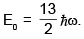• b)
If the particles are protons,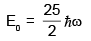• c)
If the particles are spin- less fermions,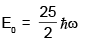• d)
If the particles are bosons,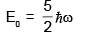Consider a one- dimensional harmonic oscillator of angular frequency &...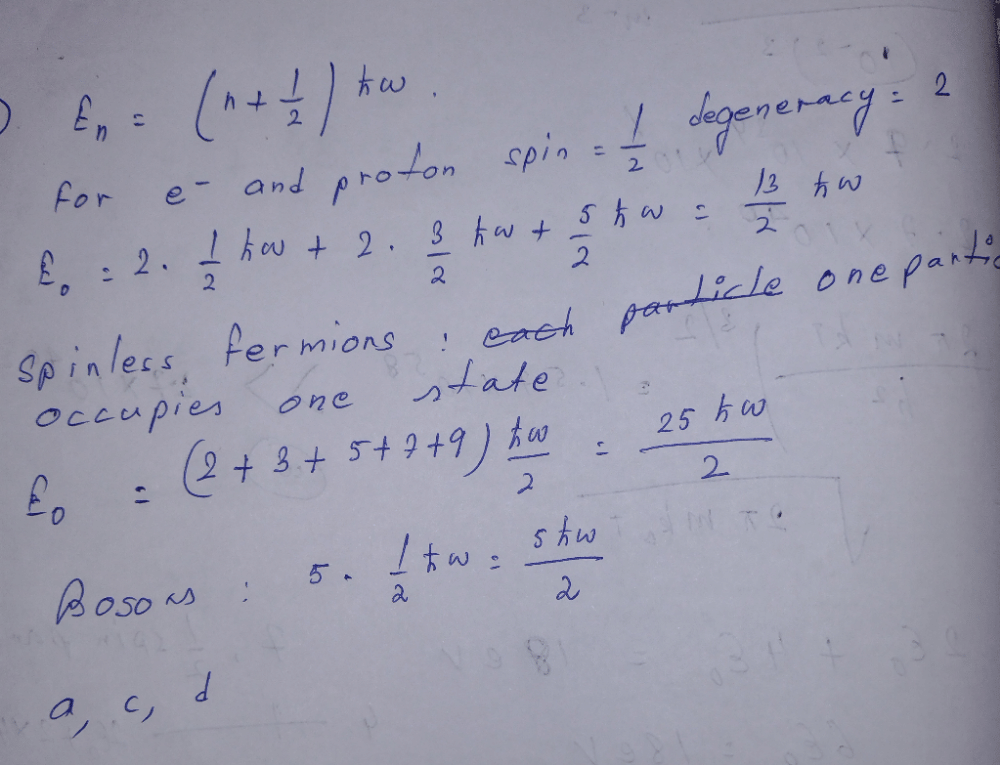1 Crore+ students have signed up on EduRev. Have you?
1 Crore+ students have signed up on EduRev. Have you?

### Learn this topic in detailPhysics - 2017 Past Year Paper 60 Ques | 180 MinsView courses related to this question

### Quick links for IIT JAM exam850+
Video Lectures2500+
Revision Notes600+
Online Tests34+
Courses
Consider a one- dimensional harmonic oscillator of angular frequency ω. If 5 identical particles occupy the energy levels of this oscillator at zero temperature, which of the following statement(s) about their ground state energy E0 is(are) correct ?a)If the particles are electrons, b)If the particles are protons, c)If the particles are spin- less fermions, d)If the particles are bosons, Correct answer is option 'A,C,D'. Can you explain this answer?
Question DescriptionExplore Courses for IIT JAM exam